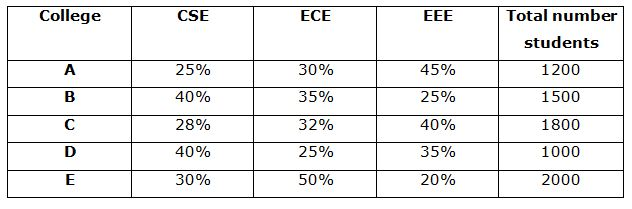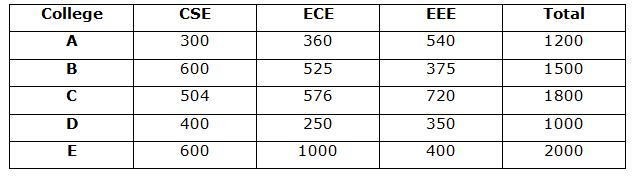# IBPS / LIC Clerk Prelims Quantitative Aptitude Questions 2019 (Day-01)

Dear Aspirants, Our IBPS Guide team is providing new series of Quantitative Aptitude Questions for IBPS Clerk Prelims 2019 so the aspirants can practice it on a daily basis. These questions are framed by our skilled experts after understanding your needs thoroughly. Aspirants can practice these new series questions daily to familiarize with the exact exam pattern and make your preparation effective.

Check here for IBPS Clerk Prelims Mock Test 2019

##### Check here for IBPS Clerk  Mock Test 2019
[WpProQuiz 7189]

Directions (1 – 5): In the following questions, two equations I and II are given. You have to solve both equations and give answer as,

a) If x > y

b) If x ≥ y

c) If x < y

d) If x ≤ y

e) If x = y or the relation cannot be established.

1)

I) x2 – 28x + 171=0

II) y2 + 3y – 108=0

2)

I) 2x2 + 18x + 40=0

II) 3y2 + 17y + 10=0

3)

I) x2 + 29x + 208=0

II) y2 + 27y + 176=0

4)

I) x2 + 22x + 112=0

II) y2 + 15y + 56=0

5)

I) 2x2 + 14x + 12=0

II) y2 – 18y + 65=0

Direction (6 – 10): Study the following information carefully and answer the given questions:

The given table shows the percentage of students in various departments of five different colleges.6) What is the difference between the total number of students from CSE departments in all the colleges together and the total number of students in college E?

a) 392

b) 404

c) 411

d) 428

e) None of these

7) What is the average number of students from EEE departments in all the colleges together?

a) 465

b) 472

c) 477

d) 480

e) None of these

8) The number of students from ECE departments in college D is what percentage of number of students from ECE departments in college E?

a) 20%

b) 25%

c) 30%

d) 35%

e) None of these

9) What is the ratio of the number of students from ECE departments in college A and B together to total number of students from college B?

a) 34:99

b) 48:99

c) 53:100

d) 59:100

e) None of these

10) What is average of the number of students from ECE in college C, the number of students from EEE in college B and the number of students from CSE in college E?

a) 515

b) 516

c) 517

d) 518

e) None of these

Directions (1-5) :

x2 – 28x + 171=0

x2 – 19x – 9x + 171=0

x(x – 19) – 9(x – 19)=0

(x – 9)(x – 19)=0

X=9, 19

y2 + 3y – 108=0

y2 + 12y – 9y – 108=0

y(y + 12) – 9(y + 12)=0

(y – 9)(y + 12)=0

Y=9, -12

2x2 + 18x + 40=0

2x2 + 10x + 8x + 40=0

2x(x + 5) + 8(x + 5)=0

(2x + 8)(x + 5)=0

x=-4, -5

3y2 + 17y + 10=0

3y2 + 15y + 2y + 10=0

3y(y + 5) + 2(y + 5)=0

(3y + 2) (y + 5)=0

Y=-2/3, -5

x2 + 29x + 208=0

x2 + 13x + 16x + 208=0

x(x + 13) + 16(x + 13)=0

(x + 16) (x + 13)=0

X=-16, -13

y2 + 27y + 176=0

y2 + 11y + 16y + 176=0

y(y + 11) + 16(y + 11)=0

(y + 16) (y + 11)=0

Y=-16, -11

x2 + 22x + 112=0

x2 + 14x + 8x + 112=0

x(x + 14) + 8(x + 14)=0

(x + 8) (x + 14)=0

X=-8, -14

y2 + 15y + 56=0

y2 + 8y + 7y + 56=0

y(y + 8) + 7(y + 8)=0

(y + 7) (y + 8)=0

Y=-7, -8

2x2 + 14x + 12=0

2x2 + 12x + 2x + 12=0

2x(x + 6) + 2(x + 6)=0

(2x + 2) (x + 6)=0

X=-2, -6

y2 – 18y + 65=0

y2 –13y – 5y + 65=0

y(y – 13) – 5(y – 13)=0

(y – 5)(y – 13)=0

Y=5, 13

Directions  (6 – 10):Total CSE students=300 + 600 + 504 + 400 + 600=2404

Total students in E=2000

Difference=2404 – 2000=404

Average=(540 + 375 + 720 + 350 + 400)/5=477

Required percentage = 250/1000 * 100=25%

Required ratio=(360 + 525) : 1500

=885:1500=177:300=59:100